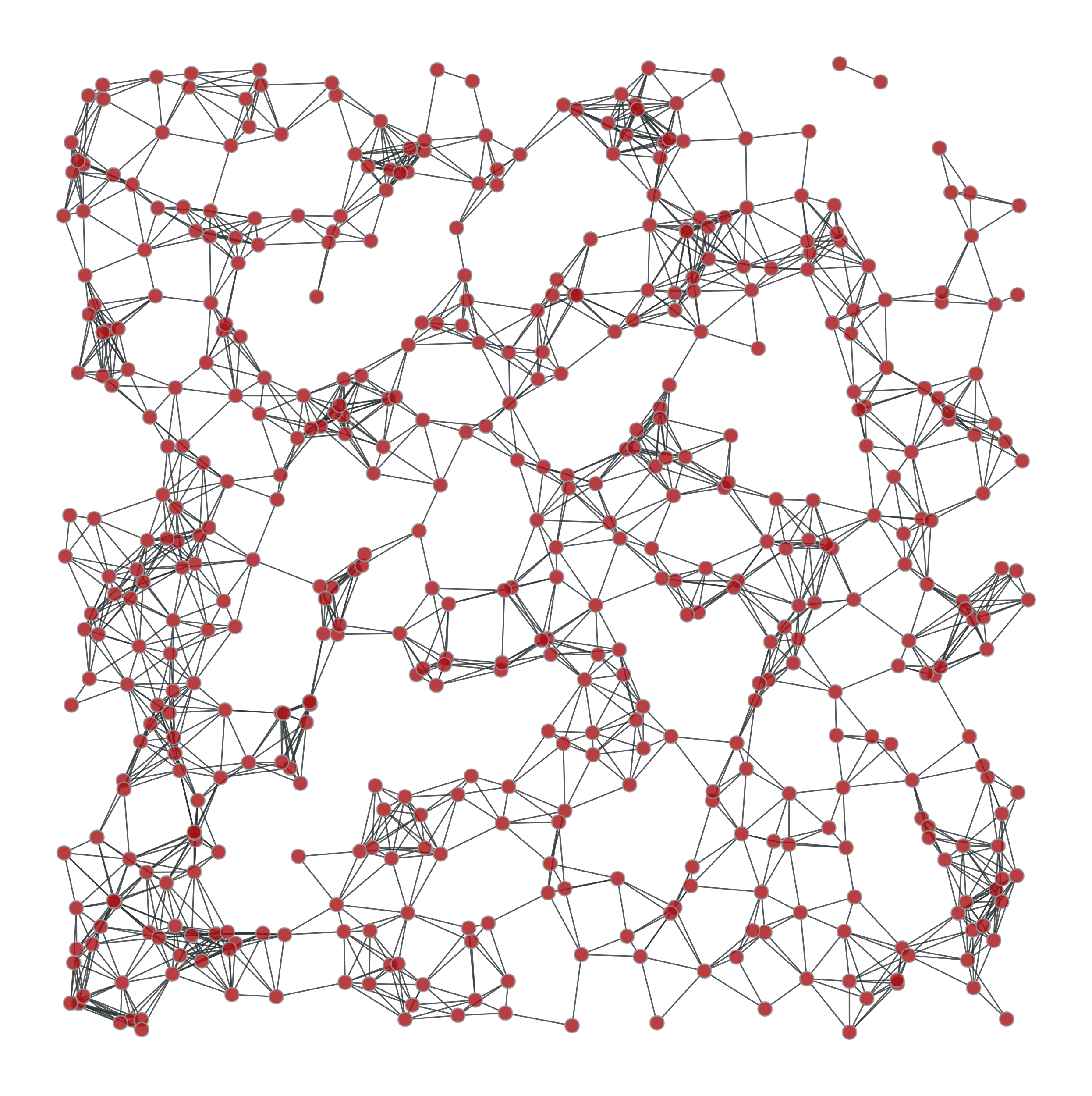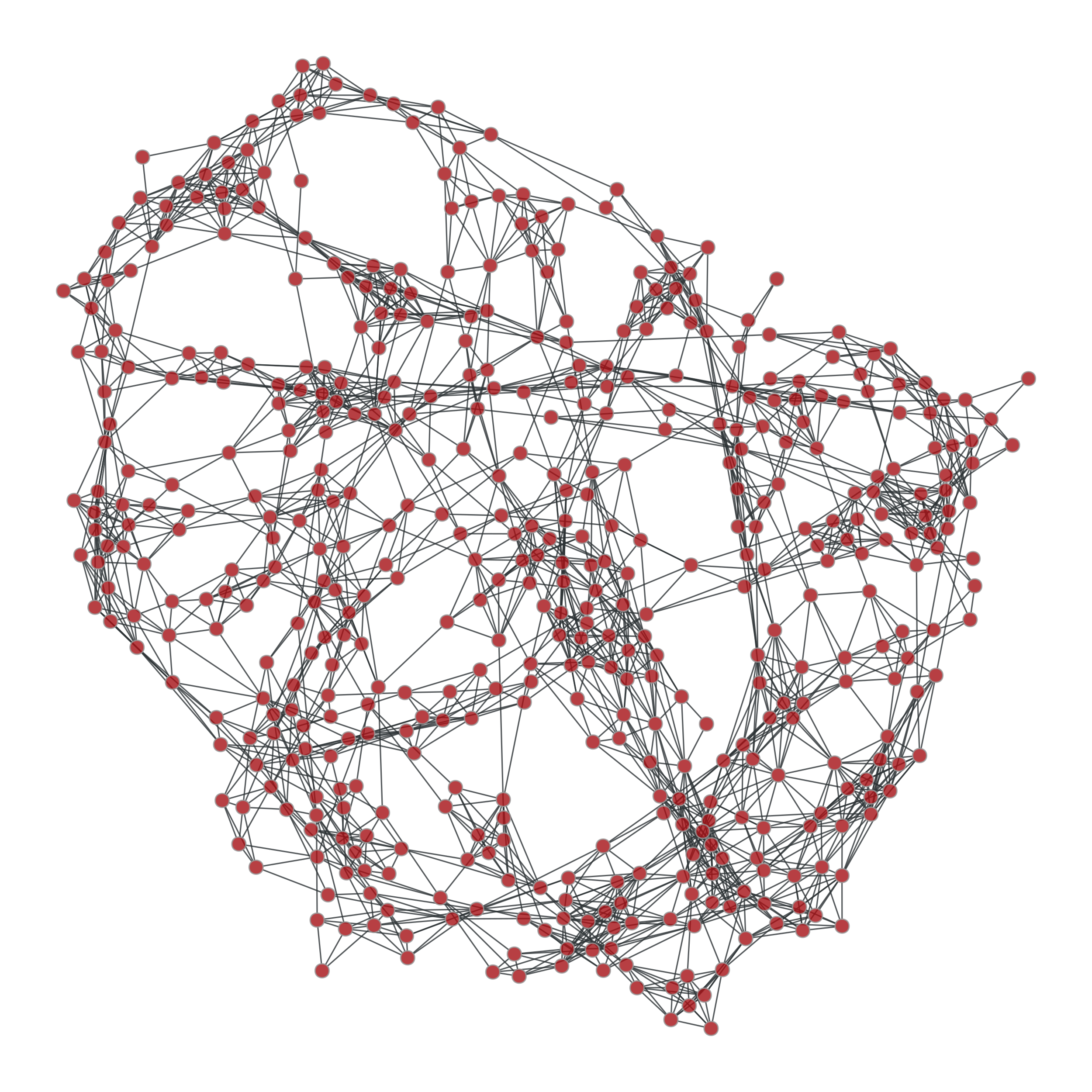# graph_tool.generation.geometric_graph#

Generate a geometric network form a set of N-dimensional points.

Parameters:
pointslist or `numpy.ndarray`

List of points. This must be a two-dimensional array, where the rows are coordinates in a N-dimensional space.

Pairs of points with an euclidean distance lower than this parameters will be connected.

rangeslist or `numpy.ndarray` (optional, default: `None`)

If provided, periodic boundary conditions will be assumed, and the values of this parameter it will be used as the ranges in all dimensions. It must be a two-dimensional array, where each row will cointain the lower and upper bound of each dimension.

Returns:
geometric_graph`Graph`

The generated graph.

pos`VertexPropertyMap`

A vertex property map with the position of each vertex.

`triangulation`

2D or 3D triangulation

`random_graph`

random graph generation

`lattice`

N-dimensional square lattice

Notes

A geometric graph [geometric-graph] is generated by connecting points embedded in a N-dimensional euclidean space which are at a distance equal to or smaller than a given radius.

References

Jesper Dall and Michael Christensen, “Random geometric graphs”, Phys. Rev. E 66, 016121 (2002), DOI: 10.1103/PhysRevE.66.016121 [sci-hub, @tor]

Examples

```>>> points = random((500, 2)) * 4
>>> g, pos = gt.geometric_graph(points, 0.3)
>>> gt.graph_draw(g, pos=pos, output="geometric.pdf")
<...>
```
```>>> g, pos = gt.geometric_graph(points, 0.3, [(0,4), (0,4)])
>>> pos = gt.graph_draw(g, output="geometric_periodic.pdf")
```Left: Geometric network with random points. Right: Same network, but

with periodic boundary conditions.